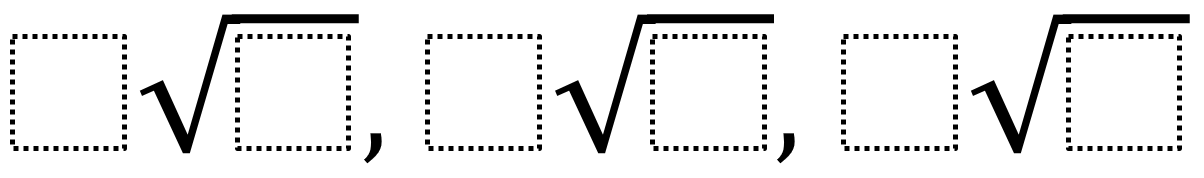Directions: Using the digits 1-9 at most one time each, create a sequence that is in numerical order and cannot be simplified anymore.### Hint

How are radicals simplified to get the index? What does that index represent?

More than one answer. One possible is 3sqr(7), 8sqr(2), 5sqr(6)

The reasoning and approach would be of real interest and a comparison amongst student answers.

Source: Phillip Haislip-Hansberry

## Imaginary Solutions to a Quadratic Equation

Directions: Using the digits 1 to 9 at most one time each, place a digit …

1.•